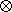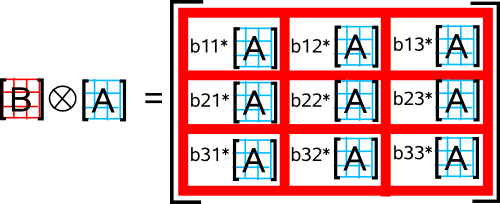# Maths - Tensor Operations

To give us quantities to work with vectors can be represented by a linear combination of basis vectors, so vector 'a' could be represented by:

a = a1 e1 + a2 e2 + a3 e3 ...

similarly vector 'b', in the same coordinate system, could be represented by:

b = b1 e1 + b2 e2 + b3 e3 ...

where:

• a and b = vectors being defined
• a1 ,a2 , a3 , b1 ,b2and b3 = scalar values
• e1 , e2 and e3= common vector bases

## Inner Product

We have already covered the dot product '•' for vectors here. This concept can be extended to tensors.

### Inner Product of Vectors

So using the above values we have:

ab = (a1 e1 + a2 e2 + a3 e3) • (b1 e1 + b2 e2 + b3 e3)

If we assume that the bases are orthogonal (mutually perpendicular), then, a dot product of a base with another will be zero except a base with itself which will be one:

e1 • e1 + e2 • e2 + e3 • e3 = 1
e1 • e2 + e2 • e3 + e3 • e1 = ... = 0

Which gives the result:

ab = a1 b1 + a2 b2 + a3 b3

#### Equivalent Matrix Equation

aT b

 a1 a2 a3
 b1 b2 b3
=
 a1 b1 + a2 b2 + a3 b3

### Inner Product of Covectors

ab = (a1 e1 + a2 e2 + a3 e3) • (b1 e1 + b2 e2 + b3 e3)

So what is the product covector bases?

e1 • e1 = ?
e1 • e2 = ?

#### Equivalent Matrix Equation

a bT

 a1 a2 a3
 a1 a2 a3
=
 a1*b1 a2*b1 a3*b1 a1*b2 a2*b2 a3*b2 a1*b3 a2*b3 a3*b3

Vector times covector:

 b1 b2 b3
 a1 a2 a3
=
 a1•b1 a2•b1 a3•b1 a1•b2 a2•b2 a3•b2 a1•b3 a2•b3 a3•b3

## Tensor Product

This denoted by, it is a more general case of the Kronecker product, it takes the right hand operand and duplicates it in every element of the left hand operand. Each of these copies is scalar multiplied by the corresponding element.The tensor product may increase the dimension or the rank of the result depending whether the multiplicands have common or independent bases.

In the above example, both the operands are rank 2 and they both use the same bases, if the operands are 3×3 matrices the result is a 9×9 matrix. So, if we use the same bases, a matrix of dimension m by na matrix of dimension u by v will give a resultant matrix of dimension m*u by n*v.

In the case below, the two vectors have different bases, shown as a column vectorrow vector which gives a matrix:

 b1 b2 b3a1 a2 a3
=
 a1*b1 a2*b1 a3*b1 a1*b2 a2*b2 a3*b2 a1*b3 a2*b3 a3*b3

So if we have independent basis vectors, say ei,ej and ek so the result will contain all the bases in all the operands, eijek=eijk

In other words, if all the bases of the operands are independent, the rank of the result will be the sum of the ranks of the operands.

So in terms of the vectors defined at the top of the page, we have:

ab = (a1 e1 + a2 e2)(b1 e1 + b 2 e2)
ab = a1*b1 (e1e1) + a1*b2 (e1e2) + a2*b1 (e2e1) + a2*b2 (e2e2)

### Other Tensor Products

#### Inner tensor product or contraction

by a vector decreases the rank of a tensor

#### Outer tensor product

by a vector increases the rank of tensors.

### Wedge Product

#### Two dimentional case

As we did for the tensor product we can take the vectors defined at the top of the page, and multiply them out:

a ^ b = (a1 e1 + a2 e2) ^ (b1 e1 + b 2 e2)
a ^ b = a1*b1 (e1^e1) + a1*b2 (e1^e2) + a2*b1 (e2^e1) + a2*b2 (e2^e2)

the difference from the tensor product is that the square of a vector is zero (as cross product in 3 dimensions) which gives:

e1^e1 = e2^e2 = 0

so:

a ^ b = a1*b2 (e1^e2) + a2*b1 (e2^e1)

also it anti-commutes so:

e1^e2= - e2^e1

which allows us to simplify to:

a ^ b = (a1*b2 - a2*b1)(e1^e2)

However it won't simplify any further, in other words e1^e2 cant be defined in terms of e1and e2 separately.

#### Three dimentional case

As we did for the tensor product we can take the vectors defined at the top of the page, and multiply them out:

 a ^ b = (a1 e1 + a2 e2+ a3 e3) ^ (b1 e1 + b 2 e2+ b 3 e3) a ^ b = a1*b1(e1^e1) + a1*b2(e1^e2) + a1*b3 (e1^e3) + a2*b1(e2^e1) + a2*b2(e2^e2)+ a2*b3(e2^e3) + a3*b1(e3^e1) + a3*b2(e3^e2)+ a3*b3(e3^e3)

as before:

e1^e1 = e2^e2 =e3^e3 = 0

and

eu^ev= (-1)nm ev^eu

which allows us to simplify to:

 a ^ b = (a1*b2- a2*b1)(e1^e2) + (a2*b3 - a3*b2)(e2^e3) + (a3*b1 -a1*b3 )(e3^e1)

as before this won't simplify any further, in other words e1^e2 , e2^e3
and e3^e1cant be defined in terms of e1, e2 and e3 separately.

## Triple Product

If

t = u • (v × w)

then in tensor notation:

t = ui eijk vj wk = eijk ui vj wk

 metadata block see also: Correspondence about this page Book Shop - Further reading. Where I can, I have put links to Amazon for books that are relevant to the subject, click on the appropriate country flag to get more details of the book or to buy it from them.Schaum's Outline of Theory and Problems of Tensor Calculus - I'm finding this hard going, it starts off with as review of linear algebra, matrix notation, etc. It redefines a lot of conventions which are hard to relearn, such as superscrips instead of subscripts to identify elements, and a summation convention, then it goes into coordinate transformations. I cant find any definition of what a tensor is. I think this book is aimed at people who already have some knowledge of the subject. I can't find any mention in this book of the term hyper-matrix. Specific to this page here:

This site may have errors. Don't use for critical systems.